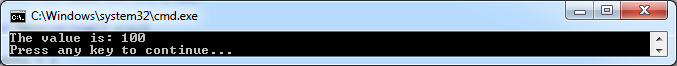# Exploring OpCodes with DynamicMethod and looking at the evaluation stack

## Posted by Filip Ekberg on 16 Oct 2011

In the previous post we looked at how our `DynamicMethod` could pass a value to another method, let's take a look at something a little bit more interesting! Consider that I want to have a method that takes an integer and this integer is manipulated and then printed out by this method. In this case, the manipulation is a multiplication and the second method is just a method to print the result in a nicely formatted way.

This is what we have, from the previous post. First of all we got the method that prints it in a formatted way, I've renamed it for clarity in this post:

``````public static void PrintWithSpecificFormat(int a)
{
Console.WriteLine("The value is: {0}", a);
}
``````

The second thing we have is the `DynamicMethod`, however, I've made two changes here, instead of taking zero parameters, I am now taking an integer parameter, secondly I've renamed it to something more understandable:

``````Type[] methodArguments = {
typeof(int)
};

var mathOperation = new DynamicMethod(
typeof(void),
methodArguments,
typeof(Program).Module);

ILGenerator il = mathOperation .GetILGenerator();
var methods = typeof(Program).GetMethods();
``````

Now let's start off by looking at the operations that we are going to emit. First of all we need to load the argument that we pass onto the evaluation stack this is done by `OpCodes.Ldarg_0`. Before we look at the other operations, notice that I mentioned the evaluation stack here, from now on it's important to understand a little bit about the evaluation stack. Values are pushed and popped onto the evaluation stack and certain operations except values to already be on the evaluation stack.

If you don't know what a stack is, here's a quick little intro with a bit of help from our friend Wikipedia. First of all, this is what a stack might look like when represented on a paper:As you can see, elements that are pushed onto the stack, is "stacked" on top of the old values and when you want to pop something, you always take out the last item that was added. This is called LIFO which stands for Last In First Out.

So if we now apply this knowledge and start thinking about how the evaluation stack works and why it's important you might see that operations such as `OpCodes.Mul` expects there to be two values that it can pop from the stack then when it's popped them, the values are multiplied and the result is pushed back onto the evaluation stack for the caller to use.

This is what it looks like when we want to multiply 10 by 20

First the value 10 is pushed onto the stack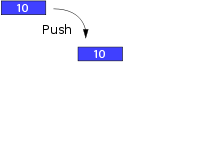Then the value 20 is pushed onto the stack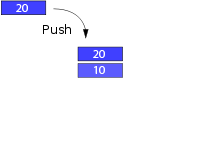Then we call `OpCodes.Mul` and it starts off by popping the first value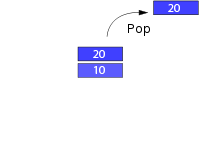The it pops the second value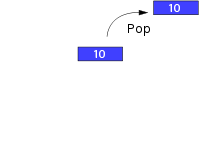After the multiplication is done, the result is pushed onto the stack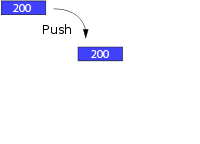This is the general pattern used, things are pushed onto the evaluation stack and then operations pops the values they want to use and then pushes a possible result back onto the evaluation stack. So how do we proceed now?

We already got the first value on the evaluation stack, let's say that whatever we pass into our dynamic method will be multiplied by 10, since we did `OpCodes.Ldarg_0` we only need to emit code that adds our next value!

This is done exactly as we did in the last post:

``````il.Emit(OpCodes.Ldc_I4, 10);
``````

If the parameter sent to the dynamic method is 20 now, the stack will look like this: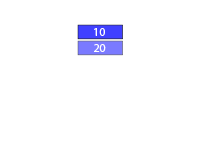Now let's do the multiplication, this is done by emitting `OpCodes.Mul`:

``````il.Emit(OpCodes.Mul);
``````

So what now? We want to pass the result from the multiplication operation to the `PrintWithSpecificFormat` method and how do we pass values again?

By pushing them to the evaluation stack!, but wait a minute, the result is already on the evaluation stack so we don't need to do anything! We can just call the method as we like. The complete IL-emitting looks like this:

``````il.Emit(OpCodes.Ldarg_0);
il.Emit(OpCodes.Ldc_I4, 10);
il.Emit(OpCodes.Mul);
il.Emit(OpCodes.Call, methods.First(x => x.Name == "PrintWithSpecificFormat"));
il.Emit(OpCodes.Ret);
``````

Now the last thing we are going to do is creating the delegate and invoking it, we can use `Action<T>` as we did in the last post and then we just invoke the created delegate:

``````var toInvoke = (Action<int>)mathOperation.CreateDelegate(typeof(Action<int>));
toInvoke(10);
``````

The result should look like this: